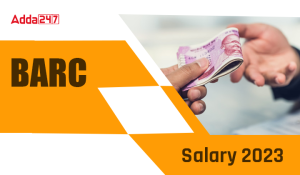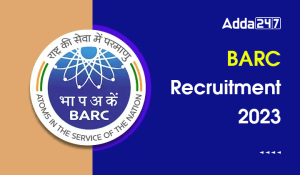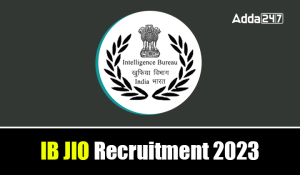Engineering Jobs   »   Mechincal Engineering quizs   »   Mechanical Engineering Quiz

# UPSSSC JE’21 ME: Daily Practices Quiz. 16-Sep-2021

Quiz: Mechanical Engineering
Exam: UPSSSC
Topic: Industrial Engg.

Each question carries 1 mark
Negative marking: 1/4 mark
Time: 8 Minutes
Q1. Breakeven point (BEP) indicates that it
(a) Recover fixed cost
(b) Recover variable cost
(c) Recover both of above costs
(d) Recover fixed, variable costs and margin of profit.

Q2. Which of the following statement is correct, when “we increase economic order quantity” then?
(a) Inventory carrying cost increases
(b) Ordering cost decreases
(c) Total cost decreases
(d) Total cost first decreases and then increases

Q3. In inventory control, the factors on which the EOQ depends on
(a) Ordering cost
(b) Holding cost
(c) Consumption rate
(d) All of these

Q4. In an Inventory control, the annual demand for a product is 400. Ordering cost for that product per order is Rs. 144 and carrying cost is Rs. 8 per unit per year. The economic lot size is
(a) 120
(b) 300
(c) 240
(d) 400

Q5. Delphi technique is used in
(a) Forecasting
(b) Inventory management
(c) Quality planning
(d) Material handling

Q6. The most commonly used method for measuring forecast error is
(a) mean absolute deviation
(b) mean absolute deviation percentage error
(c) mean standard deviation error
(d) mean square error

Solutions
S1. Ans. (c)
Sol. Breakeven point (BEP) indicates that it recovers fixed cost and variable cost.
S2. Ans. (d)
Sol. When we increase economic order quantity, then the total cost first decreases and then increases. As shown in the graph.Order Size
S3. Ans. (d)
Sol. In inventory control, the factors on which EOQ depends on
Holding cost
Ordering cost
Consumption rate
EOQ=(2DC_o)/C_h
S4. Ans. (a)
Sol. As we know that
EOQ=(2DC_o)/C_h
Where,
D=Annual Demand=400
C_o=Ordering cost=Rs.144 per order
C_h=Holding cost=Rs.8 per unit per year
EOQ=(2×400×144)/8=120
S5. Ans. (a)
Sol. Delphi technique: The Delphi technique is used in forecasting and it is used as group opinions of the panel of experts on the problem being encountered.
S6. Ans. (d)
Sol. The most commonly used method for measuring forecast error is mean square error.
MSE=(∑▒〖(D_t 〗-F_t)²)/N

Sharing is caring!

•GATE Result 2023 Out, Download Result, C...
•GATE 2023 Admit Card Out, Download Link ...
•BARC Previous Year Question Papers, Down...
•BARC Salary 2023, Check Perks and Allowa...
•BARC Recruitment 2023, Notification, Vac...
•ISRO Scientist Recruitment 2023 Out, App...
•IB JIO Recruitment 2023 Out, Direct Link...
•DFCCIL Vacancy 2023 Out, Apply Online fo...